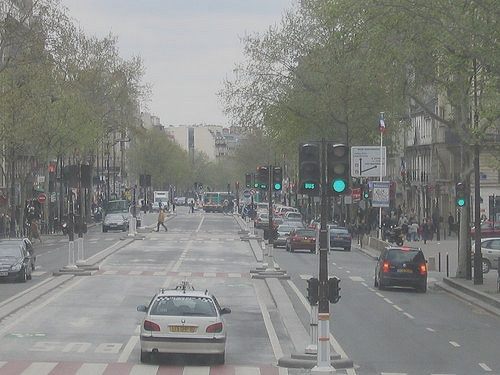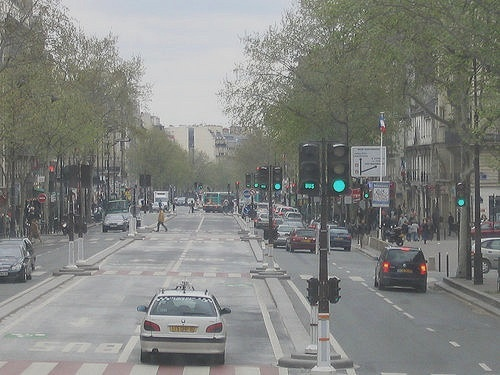# Normalizing an image in OpenCV Python

Fellow coders, in this tutorial we will normalize images using OpenCV’s “cv2.normalize()” function in Python. Image Normalization is a process in which we change the range of pixel intensity values to make the image more familiar or normal to the senses, hence the term normalization. Often image normalization is used to increase contrast which aids in improved feature extraction or image segmentation.

Often times Image Normalization is used to remove noise from the picture (data). With the help of Image Normalization, we can remove high-frequency noise and very low noise from the image which is really helpful. When we look at an image that is unclear to our senses, it becomes stressful for our eyes. But with the help of Image Normalization, we bring the image into a range of intensity values which is normal to our senses and hence we do not have to stress our visual senses and can clearly understand what is going on in an image. Whenever an image gets a poor contrast due to any reason our aim is to fix the contrast of the image so that it is normal to our senses.

In a normalized image:

• Mean = 0
• Variance = 1

## Working with the code: Normalize an image in Python with OpenCV

Input Image:```import cv2 as cv
import numpy as np

norm_img = np.zeros((800,800))
final_img = cv.normalize(img,  norm_img, 0, 255, cv.NORM_MINMAX)
cv.imshow('Normalized Image', final_img)
cv.imwrite('city_normalized.jpg', final_img)
cv.waitKey(0)
cv.destroyAllWindows()```

We can also use cv.NORM_INF, cv.NORM_L1 or cv.NORM_L2 in place of cv.NORM_MINMAX.

Output Image:We can clearly see that in the output image, contrast is increased and the image looks better.

Also read: Bilateral Filter in OpenCV in Python